# iqimbal

Apply I/Q imbalance to input signal

## Syntax

``y = iqimbal(x,A)``
``y = iqimbal(x,A,P)``

## Description

example

````y = iqimbal(x,A)` applies I/Q amplitude imbalance `A` to input signal `x`.```

example

````y = iqimbal(x,A,P)` applies I/Q amplitude imbalance `A` and phase imbalance `P` to input signal `x`. ```

## Examples

collapse all

Generate a 16-QAM signal. Display the scatter plot.

```x = qammod(randi([0 15],1000,1),16); h = scatterplot(x); hold on```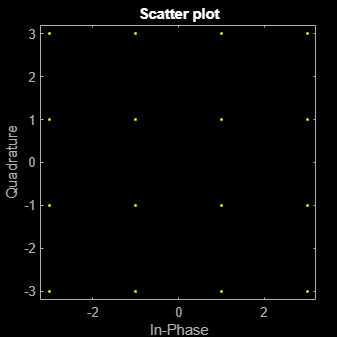Apply a 10 dB amplitude imbalance. A positive amplitude imbalance causes horizontal stretching of the constellation.

```y = iqimbal(x,10); scatterplot(y,1,0,'ro',h)```Apply a -10 dB amplitude imbalance. A negative amplitude imbalance causes vertical stretching of the constellation.

```z = iqimbal(x,-10); scatterplot(z,1,0,'k*',h) hold off```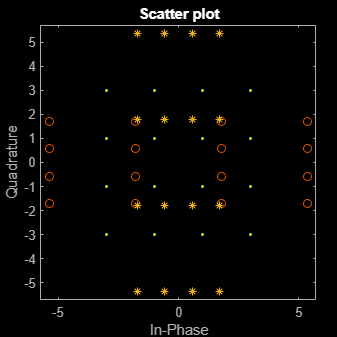Generate a 16-QAM signal having two channels.

`x = qammod(randi([0 15],1000,2),16);`

Apply a 3 dB amplitude imbalance and a 10 degree phase imbalance to the first channel. Apply a –5 dB amplitude imbalance and a –15 degree phase imbalance to the second channel.

`y = iqimbal(x,[3 -5],[10 -15]);`

Plot the constellation diagram of both channels of the impaired signal.

```h = scatterplot(y(:,1),1,0,'b*'); hold on scatterplot(y(:,2),1,0,'ro',h) hold off```The first channel is stretched horizontally, and the second channel is stretched vertically.

Apply a 1 dB, 5 degree I/Q imbalance to a QPSK signal. Then apply a DC offset. Visualize the offset using a spectrum analyzer.

Generate a QPSK sequence.

```x = pskmod(randi([0 3],1e4,1),4,pi/4); ```

Apply a 1 dB amplitude imbalance and 5 degree phase imbalance to a QPSK signal. Apply a 0.5 + 0.3i DC offset.

```y = iqimbal(x,1,5); z = y + complex(0.5,0.3); ```

Plot the spectrum of the impaired signal.

```sa = dsp.SpectrumAnalyzer('SampleRate',1000,'YLimits',[-50 30]); sa(z) ```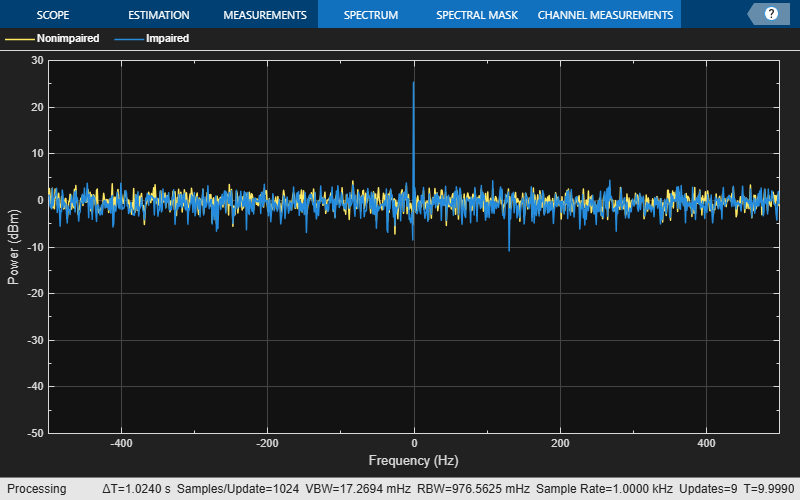Display the corresponding scatter plot.

```scatterplot(z) grid ```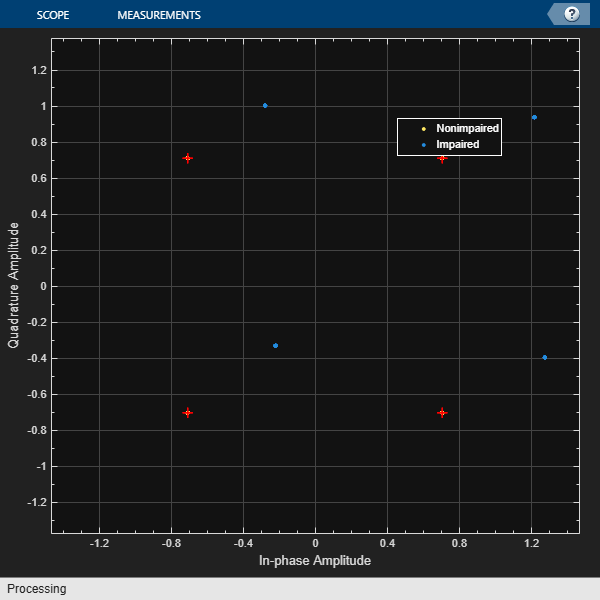The effect of the I/Q imbalance and the DC offset is observable.

Generate random data and apply 8-PSK modulation.

```data = randi([0 7],2000,1); txSig = pskmod(data,8,pi/8); ```

Pass the transmitted signal through an AWGN channel. Apply an I/Q imbalance.

```noisySig = awgn(txSig,20); rxSig = iqimbal(noisySig,2,20); ```

Create a constellation diagram object that displays only the last 1000 symbols. Plot the constellation diagram of the impaired signal.

```cd = comm.ConstellationDiagram('ReferenceConstellation',pskmod(0:7,8,pi/8), ... 'SymbolsToDisplaySource','Property','SymbolsToDisplay',1000); cd(rxSig) ```Correct for the I/Q imbalance by using a `comm.IQImbalanceCompensator` object. Plot the constellation diagram of the signal after compensation.

```iqComp = comm.IQImbalanceCompensator('StepSize',1e-3); compSig = iqComp(rxSig); cd(compSig) ```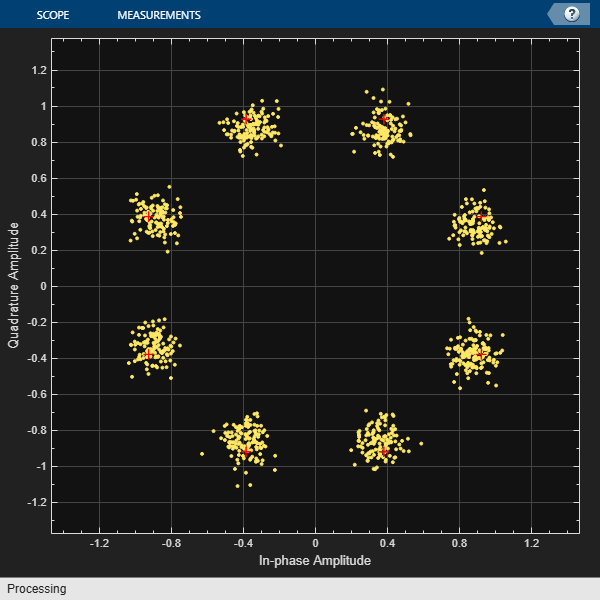The compensator removes the I/Q imbalance.

## Input Arguments

collapse all

Input signal, specified as a column vector or matrix. The function supports multichannel operations, where the number of columns corresponds to the number of channels.

Example: `pskmod(randi([0 3],100,1),4,pi/4)`

Data Types: `single` | `double`
Complex Number Support: Yes

Amplitude imbalance in dB, specified as a real scalar or row vector.

• If `A` is a scalar, the function applies the same amplitude imbalance to each channel.

• If `A` is a vector, then each element specifies the amplitude imbalance that is applied to the corresponding column (channel) of the input signal. The number of elements in `A` must equal the number of columns in `x`.

Example: `3`

Example: ```[0 5]```

Data Types: `single` | `double`

Phase imbalance in degrees, specified as a real scalar or row vector.

• If `P` is omitted, a phase imbalance of zero degrees is used.

• If `P` is a scalar, the function applies the same phase imbalance to each channel.

• If `P` is a vector, then each element specifies the phase imbalance that is applied to the corresponding column (channel) of the input signal. The number of elements in `P` must equal the number of columns in `x`.

Example: `10`

Example: ```[2.5 7]```

Data Types: `single` | `double`

## Output Arguments

collapse all

Output signal, returned as a vector or matrix having the same dimensions as `x`. The number of columns in `y` corresponds to the number of channels.

Data Types: `single` | `double`
Complex Number Support: Yes

## Algorithms

The `iqimbal` function applies an I/Q amplitude and phase imbalance to an input signal.

Given amplitude imbalance Ia in dB, the gain, g, resulting from the imbalance is defined as

`$g\triangleq {g}_{r}+i{g}_{i}=\left[{10}^{0.5\frac{{I}_{a}}{20}}\right]+i\left[{10}^{-0.5\frac{{I}_{a}}{20}}\right].$`

Applying the I/Q imbalance to input signal x results in output signal y such that

`$y=\mathrm{Re}\left(x\right)\cdot {g}_{r}{e}^{-i0.5{I}_{p}\left(\pi /180\right)}+i\mathrm{Im}\left(x\right)\cdot {g}_{i}{e}^{i0.5{I}_{p}\left(\pi /180\right)}\text{\hspace{0.17em}},$`

where g is the imbalance gain and Ip is the phase imbalance in degrees.

## Version History

Introduced in R2016b